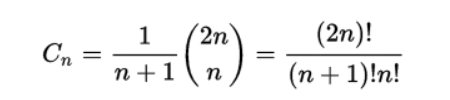# 栈

## 栈的定义

InitStack(&S)：初始化一个空栈S。

StackEmpty(S)：判断一个栈是否为空，若栈为空则返回true，否则返回false。

Push(&S, x)：进栈，若栈S未满，则将x加入使之成为新栈顶。

Pop(&S, &x)：出栈，若栈非空，则弹出栈顶元素，并用x返回。

GetTop(S, &x)：读栈顶元素，若栈非空则用x返回栈顶元素。

ClearStack(&S)：销毁栈，并释放S占用的内存空间

## 顺序栈

``````# define MaxSize 50
typedef struct{
ElemType date[MaxSize];
//top用来存放栈顶位置
int top;
}SqStack;
``````

``````void TnitStack (SqStack &S){
S.top=-1;
}
``````

``````bool StackEmpty(SqStack S){
if(S.top==-1)
return true;
else
return false;
}
``````

``````bool Push(SqStack &S,ElemType x){
if(S.top==MaxSize-1)
return false;
S.date[++S.top]=x;
return true;
}
``````

``````bool Pop(SqStack &s,ElemType &x){
if(S.top==-1)
return false;
x=S.date[S.top--];
return true;
}
``````

``````bool Pop(SqStack S,ElemType &x){
if(S.top==-1)
return false;
x=S.date[S.top];
return true;
``````

## 链栈

``````typedef struct Linknode{
ElemType date;
}*LiStack;
``````

# 队列

## 队列的定义

InitQueue(&Q)：初始化队列，构造一个空队列Q。
QueueEmpty(Q)：判队列空，若队列Q为空返回 true，否则返回false。
EnQueue(&Q, x)：入队，若队列Q未满，则将x加入使之成为新的队尾。
DeQueue(&Q, &x)：出队，若队列Q非空，则删除队头元素，并用x返回。
ClearQueue(&Q)：销毁队列，并释放队列Q占用的内存空间

## 顺序队列

``````# define MaxSize 50
typedef struct{
ElemType date[MaxSize];
int front,rear;
} SqQueue;
``````

front一般指向队头元素，rear一般指向队尾元素的下一位置。也可以front指向队头元素的前一位置，rear指向队尾元素。两种指向位置不同对应操作也不同，但是我们一般用前者，今天也是讲解前者。

### 循环队列

front指针移动：
Q.front = (Q.front + 1) % MaxSize
rear指针移动：
Q.rear = (Q.rear + 1) % MaxSize

(Q.rear + MaxSize - Q.front) % MaxSize

``````bool EnQueue(SqQueue &Q,ElemType x){
if(Q.front==(Q.rear+1)%MaxSize)
return false;
Q.date[Q.rear]=x;
Q.rear = (Q.rear + 1) % MaxSize;
return true;
}
``````

``````bool DeQueue(SqQueue &Q,ElemType &x){
if(Q.rear == Q.front)
return false;
x=Q.date[Q.front];
Q.front = (Q.front+1)%MaxSize;
return true;
}
``````

## 链式队列

``````typedef struct{
ElemType date;

typedef struct{
``````

``````void InitQueue(LinkQueue &Q){
Q,rear = Q.front;
Q.front->next=NULL;
}
``````

``````bool isEmpty(LinkQueue Q){
if(Q.front == Q.rear)
return true;
else
return false;
}
``````

``````void EnQueue(LinkQueue &Q,ElemType x){
s->date=x;
s->next=NULL;
Q->rear->next=s;
Q.rear=s;
}
``````

``````bool DeQueue(LinkQueue &Q,ElemType &x){
if(Q.rear==Q.front)
return false;
x=p->date;
Q.front->next=p->next;
if(Q.rear==p)
Q.rear=Q.front;
free(p);
return true;
}
``````

# 栈与队列的应用

1234 1243 1324 1342 1423 1432
2134 2143 2314 2341 2413 2431
3124 3142 3214 3241 3412 3421
4123 4132 4213 4231 4312 4321## 栈的应用

### 表达式求值

a. 若为’(’ ，入栈；
b. 若为’)’，则依次把栈中的运算符加入后缀表达 式，直到出现’(’，并从栈中删除’(’；
c. 若为’+’，’-’，’*’，’/’，
· 栈空，入栈；
· 栈顶元素为’(’，入栈；
· 高于栈顶元素优先级，入栈；
· 否则，依次弹出栈顶运算符，直到一个优先级比 它低的运算符或’('为止；
d. 遍历完成，若栈非空依次弹出所有元素

· 若是数字压入栈中；
· 若是操作符，连续弹出栈中两个元素，按操作符计算并把结果压入栈中。
· 扫描完毕后栈顶为最后结果

### 括号匹配

1）初始一个空栈，顺序读入括号。
2）若是右括号，则与栈顶元素进行匹配
· 若匹配，则弹出栈顶元素并进行下一个元素
· 若不匹配，则该序列不合法
3）若是左括号，则压入栈中
4）若全部元素遍历完毕，栈中非空则序列不合法

### 递归

``````int Fib(int n){
if(n==0)
return 0;
else if(n==1)
return 1;
else
return Fib(n-1)+Fib(n-2);
}
``````

· 在递归调用过程中，系统为每一层的返回点、局部变量、传入实参等开辟 了递归工作栈来进行数据存储，递归次数过多容易造成栈溢出